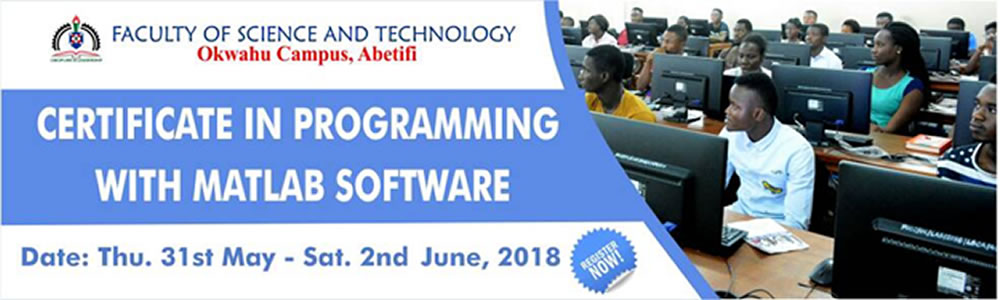This programme is uniquely designed to provide hands-on training on the use of MATLAB Software.
WHAT IS MATLAB?
MATLAB is a programming package specifically designed for quick and easy scientific calculations. It integrates computation, visualization, and programming in an easy-to-use environment where problems and solutions are expressed in familiar mathematical notation. Typical uses include:

• Data analysis, exploration, and visualization
• Modeling and simulation
• Algorithm development
• Graphical Analysis
• Application development
• Mathematical computations

MATLAB is being used in a wide variety of domains from the natural sciences, through all disciplines of engineering, to finance, and beyond, and it is heavily used in industry. Hence, a solid background in MATLAB is an indispensable skill in today’s job market.
WHO SHOULD ATTEND?
Mathematicians, Engineers, Statisticians, Mathematics Teachers, Software Developers,  Actuarial Analysts, Data Scientists/Analysts, Mathematics Research Scientists,  Postgraduate/Undergraduate Mathematics students and individuals interested in  knowing and using MATLAB Software.